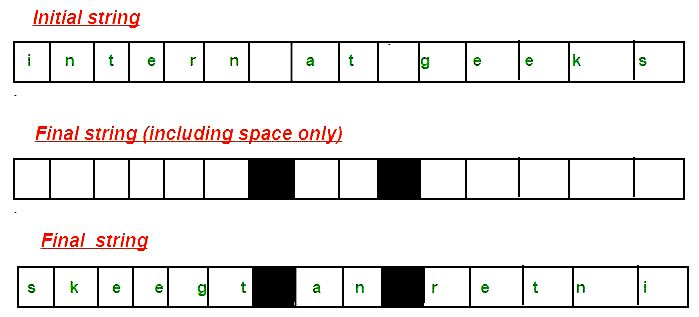# Reverse a string preserving space positions

Write a program to reverse the given string while preserving the position of spaces.
Examples:

```Input  : "abc de"
Output : edc ba

Input : "intern at geeks"
Output : skeegt an retni

Input : "Help others"
Output : sreh topleH

```1. Create a string to store results. Mark the space position of the given string in this string.
2. Insert the character from the input string into the result string in reverse order.
3. While inserting the character check if the result string already contains a space at index ‘j’ or not. If it contains, we copy the character to the next position.

Below is the implementation of the above steps.

## C++

 `// C++ program to reverse a string preserving` `// spaces.` `#include ` `using` `namespace` `std;`   `// Function to reverse the string` `// and preserve the space position` `string reverses(string str)` `{` `    ``// Mark spaces in result` `    ``int` `n = str.size();` `    ``string result(n, ``'\0'``);` `    ``for` `(``int` `i = 0; i < n; i++)` `        ``if` `(str[i] == ``' '``)` `            ``result[i] = ``' '``;`   `    ``// Traverse input string from beginning` `    ``// and put characters in result from end` `    ``int` `j = n - 1;` `    ``for` `(``int` `i = 0; i < str.length(); i++) {` `        ``// Ignore spaces in input string` `        ``if` `(str[i] != ``' '``) {` `            ``// ignore spaces in result.` `            ``if` `(result[j] == ``' '``)` `                ``j--;`   `            ``result[j] = str[i];` `            ``j--;` `        ``}` `    ``}`   `    ``return` `result;` `}`   `// Driver code` `int` `main()` `{` `    ``string str = ``"internship at geeks for geeks"``;` `    ``cout << reverses(str) << endl;` `    ``return` `0;` `}`

## Java

 `// Java program to reverse a string` `// preserving spaces.` `public` `class` `ReverseStringPreserveSpace {` `    ``// Function to reverse the string` `    ``// and preserve the space position` `    ``static` `void` `reverses(String str)` `    ``{`   `        ``char``[] inputArray = str.toCharArray();` `        ``char``[] result = ``new` `char``[inputArray.length];`   `        ``// Mark spaces in result` `        ``for` `(``int` `i = ``0``; i < inputArray.length; i++) {` `            ``if` `(inputArray[i] == ``' '``) {` `                ``result[i] = ``' '``;` `            ``}` `        ``}`   `        ``// Traverse input string from beginning` `        ``// and put characters in result from end` `        ``int` `j = result.length - ``1``;` `        ``for` `(``int` `i = ``0``; i < inputArray.length; i++) {`   `            ``// Ignore spaces in input string` `            ``if` `(inputArray[i] != ``' '``) {`   `                ``// ignore spaces in result.` `                ``if` `(result[j] == ``' '``) {` `                    ``j--;` `                ``}` `                ``result[j] = inputArray[i];` `                ``j--;` `            ``}` `        ``}` `        ``System.out.println(String.valueOf(result));` `    ``}`   `    ``// driver function` `    ``public` `static` `void` `main(String[] args)` `    ``{` `        ``reverses(``"internship at geeks for geeks"``);` `    ``}` `}`   `// This code is contributed by Rishabh Jain`

## C#

 `// C# program to reverse a` `// string preserving spaces.` `using` `System;`   `class` `GFG {`   `    ``// Function to reverse the string` `    ``// and preserve the space position` `    ``static` `void` `reverses(``string` `str)` `    ``{` `        ``char``[] inputArray = str.ToCharArray();` `        ``char``[] result = ``new` `char``[inputArray.Length];`   `        ``// Mark spaces in result` `        ``for` `(``int` `i = 0; i < inputArray.Length; i++) {` `            ``if` `(inputArray[i] == ``' '``) {` `                ``result[i] = ``' '``;` `            ``}` `        ``}`   `        ``// Traverse input string from beginning` `        ``// and put characters in result from end` `        ``int` `j = result.Length - 1;` `        ``for` `(``int` `i = 0; i < inputArray.Length; i++) {`   `            ``// Ignore spaces in input string` `            ``if` `(inputArray[i] != ``' '``) {`   `                ``// ignore spaces in result.` `                ``if` `(result[j] == ``' '``) {` `                    ``j--;` `                ``}` `                ``result[j] = inputArray[i];` `                ``j--;` `            ``}` `        ``}` `        ``for` `(``int` `i = 0; i < result.Length; i++)` `            ``Console.Write(result[i]);` `    ``}`   `    ``// Driver code` `    ``public` `static` `void` `Main()` `    ``{` `        ``reverses(``"internship at geeks for geeks"``);` `    ``}` `}`   `// This code is contributed by Sam007`

Output:

```skeegrofsk ee gtapi hsn retni

```

Time complexity -> O(N)
Auxiliary Space -> O(N)
Optimized Solution
The idea is to use two pointers to reverse.

## C++

 `#include ` `using` `namespace` `std;`   `void` `preserveSpace(string &str)` `{` `    ``int` `n = str.length();`   `    ``// Initialize two pointers as two corners` `    ``int` `start = 0;` `    ``int` `end = n - 1;`   `    ``// Move both pointers toward each other` `    ``while` `(start < end) {`   `        ``// If character at start or end is space,` `        ``// ignore it` `        ``if` `(str[start] == ``' '``) {` `            ``start++;` `            ``continue``;` `        ``}` `        ``else` `if` `(str[end] == ``' '``) {` `            ``end--;` `            ``continue``;` `        ``}`   `        ``// If both are not spaces, do swap` `        ``else` `{` `            ``swap(str[start], str[end]);` `            ``start++;` `            ``end--;` `        ``}` `    ``}` `}`   `// Driver code` `int` `main()` `{` `    ``string str = ``"internship at geeks for geeks"``;` `    ``preserveSpace(str);` `    ``cout << str;` `    ``return` `0;` `}`

## Python3

 `# Python3 program to implement` `# the above approach` `def` `preserveSpace(``Str``):` `  `  `    ``n ``=` `len``(``Str``)` `    ``Str` `=` `list``(``Str``)`   `    ``# Initialize two pointers ` `    ``# as two corners ` `    ``start ``=` `0` `    ``end ``=` `n ``-` `1`   `    ``# Move both pointers ` `    ``# toward each other ` `    ``while``(start < end):`   `        ``# If character at start ` `        ``# or end is space, ` `        ``# ignore it ` `        ``if``(``Str``[start] ``=``=` `' '``):` `            ``start ``+``=` `1` `            ``continue` `        ``elif``(``Str``[end] ``=``=` `' '``):` `            ``end ``-``=` `1` `            ``continue` `            `  `        ``# If both are not ` `        ``# spaces, do swap ` `        ``else``:` `            ``Str``[start], ``Str``[end] ``=` `(``Str``[end],` `                                    ``Str``[start])` `            ``start ``+``=` `1` `            ``end ``-``=` `1` `    ``print``(''.join(``Str``))`   `# Driver code ` `Str` `=` `"internship at geeks for geeks"` `preserveSpace(``Str``); `   `# This code is contributed by avanitrachhadiya2155`

Output:

```skeegrofsk ee gtapi hsn retni

```

This article is contributed by Rishabh Jain. If you like GeeksforGeeks and would like to contribute, you can also write an article using contribute.geeksforgeeks.org or mail your article to contribute@geeksforgeeks.org. See your article appearing on the GeeksforGeeks main page and help other Geeks.

Attention reader! Don’t stop learning now. Get hold of all the important DSA concepts with the DSA Self Paced Course at a student-friendly price and become industry ready.

My Personal Notes arrow_drop_up

Article Tags :

3

Please write to us at contribute@geeksforgeeks.org to report any issue with the above content.